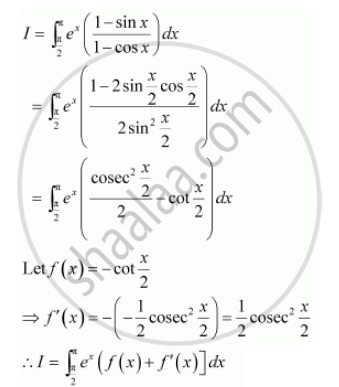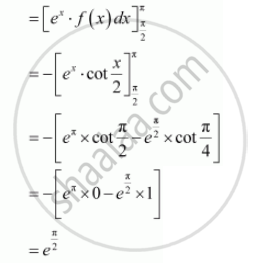Share
Notifications

View all notifications
Books Shortlist
Your shortlist is empty

# Solution for Evaluate the Definite Integrals Int_(Pi/2)^Pi E^X ((1-sinx)/(1-cos X)) Dx - CBSE (Science) Class 12 - Mathematics

Login
Create free account

Forgot password?
ConceptDefinite Integral as the Limit of a Sum

#### Question

Evaluate the definite integrals int_(pi/2)^pi e^x ((1-sinx)/(1-cos x)) dx

#### SolutionIs there an error in this question or solution?

#### Video TutorialsVIEW ALL 

Solution Evaluate the Definite Integrals Int_(Pi/2)^Pi E^X ((1-sinx)/(1-cos X)) Dx Concept: Definite Integral as the Limit of a Sum.
S